# Mapping Coronavirus with PythonCoronavirus

# Web Scraping

`#import librariesimport os, sysimport jsonimport pandas as pdimport geopandas as gpdimport numpy as npfrom numpy import int64import requests, ioimport urllib.requestimport foliumfrom folium import pluginsimport fionaimport brancafrom branca.colormap import linearfrom bs4 import BeautifulSoupimport matplotlib.pyplot as plt`
`url = ‘https://www.worldometers.info/coronavirus/#countries'response = requests.get(url)`
`data = response.content.decode(‘utf-8’)`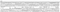Web Scraping
`hp = HTMLTableParser()table = hp.parse_url(url) # Grabbing the table from the tupletable.head(10)`
`#check bottom rowstable.tail(10)`
`#Drop top buttom unwanted rowsdf= table.drop(table.index[[0,1,2,3,4,5,6,7]]).reset_index(drop=True)#drop tail unwanted rowsdf.drop(df.tail(8).index,inplace=True)#drop new line '\n' charachter df.replace(['\n'], '', regex=True, inplace=True)df.replace([','], '', regex=True, inplace=True)`
`# drop unwanted drop unwanted special characters using a loopfor col in df.columns[1:11]:     df[col]=df[col].str.replace(“+”, “”).str.replace(“,”, “”).str.replace(“N/A”, “”).str.replace(“ “, “”).str.replace(“ “, “”)`
`df1 = df.rename(columns={'Country,Other': 'CNTRY_NAME', 'Serious,Critical': 'Serious_Critical', 'Tot Cases/1M pop': 'Tot_Cases_1M_pop', 'Deaths/1M pop': 'Deaths_1M_pop', 'Tests/\n1M pop\n': 'Tests_1M_pop'})`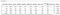Worldometer Coronavirus Table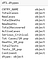Data Types
`#convert object columns in dataframe to numericdf1.fillna(0, inplace=True)df1.replace(np.nan, 0, inplace=True)df1.replace(np.inf, 0, inplace=True)for col in df1.columns[1:11]:     df1[col] = pd.to_numeric(df1[col], errors=’ignore’)`
`#convert float columns in data frame to integerdf1.fillna(0, inplace=True)df1.replace(np.nan, 0, inplace=True)df1.replace(np.inf, 0, inplace=True)for col in df1.columns[1:11]:     df1[col]=df1[col].apply(int)`
`df1.sort_values(by=[‘TotalCases’], inplace=True, ascending=False)df1.head(10)`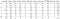Cleaned Worldometer Table

# Mapping

`# get country dataurl = "https://opendata.arcgis.com/datasets/a21fdb46d23e4ef896f31475217cbb08_1.geojson"world = gpd.read_file(url)world.plot()`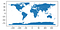Countries WGS84 (ArcGIS Hub)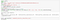Downloading Zipped Shapefile
`world.CNTRY_NAME = world.CNTRY_NAME.replace({"United States": "USA"},{"United Kingdom": "UK"})`
`corona = world.merge(df1, on='CNTRY_NAME', how='left')corona.dtypes`
`corona.fillna(0, inplace=True)corona.replace(np.nan, 0, inplace=True)corona.replace(np.inf, 0, inplace=True)for col in corona.columns[3:10]:     corona[col]=corona[col].astype(int)corona.dtypes`
`df_world = pd.merge(df1, world, on=’CNTRY_NAME’)crs = {‘init’: ‘epsg:4326’}corona_gpd = gpd.GeoDataFrame(df_world, crs=crs, geometry=’geometry’)corona_gpd.head(5)`

# Plotting Data

`f, ax = plt.subplots(1,1,figsize=(12,8))ax = corona_gpd.plot(column=’TotalCases’, cmap=’rainbow’, ax=ax, legend=True,  legend_kwds={‘label’: “Total Cases by Country” })`
`ax = corona_gpd.plot(figsize=(15, 15), column=’TotalDeaths’, cmap=plt.cm.jet, scheme=’fisher_jenks’, k=9, alpha=1, legend=True, markersize = 0.5 )plt.title(‘Coronavirus Total Death by Country’)`
`import hvplot.pandascorona_gpd.hvplot(c=”TotalDeaths”, cmap=’rainbow’,   width=800,height=450,   title=”TotalDeaths by Country”) `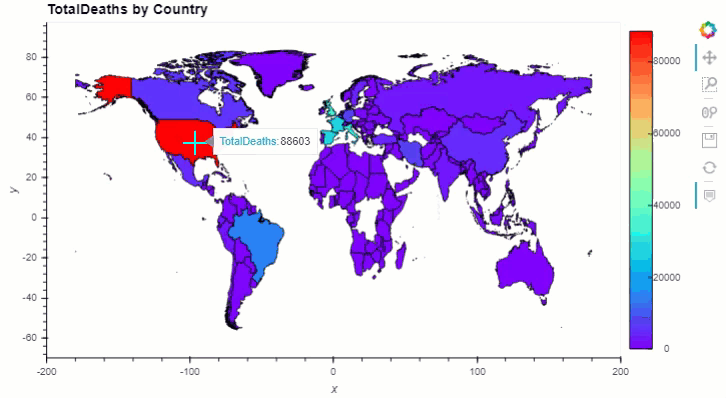Interactive Plotting
`# plotting time series datacovid= pd.read_csv('https://covid.ourworldindata.org/data/owid-covid-data.csv', delimiter= ",") covid1 = covid.rename(columns={'location': 'COUNTRY'})covid_gdp = pd.merge(world, covid1)crs = {'init': 'epsg:4326'}covid_gdp = gpd.GeoDataFrame(covid_gdp, crs=crs, geometry='geometry')covid_gdp.hvplot( c="total_deaths",                  cmap="YlOrRd",                  hover_cols=['COUNTRY', 'total_deaths'],                  hover_fill_color="grey",                  line_width=2,                  width=800,                                  height=450,                  groupby='date',                  title="Covid-19 Total Deaths by Country/Date")`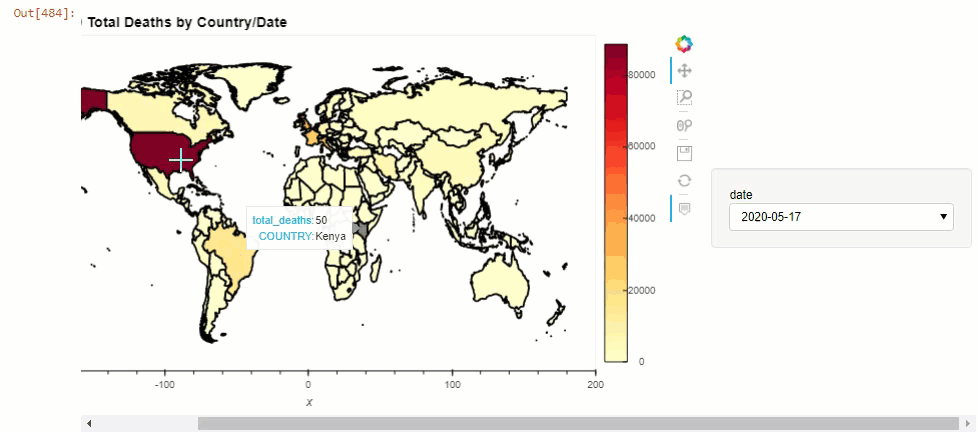Covid-19 Timeseries Data

# Interactive Mapping

`import folium #OSM Mapm = folium.Map(location=[43.6532, -79.3832])#Torontom`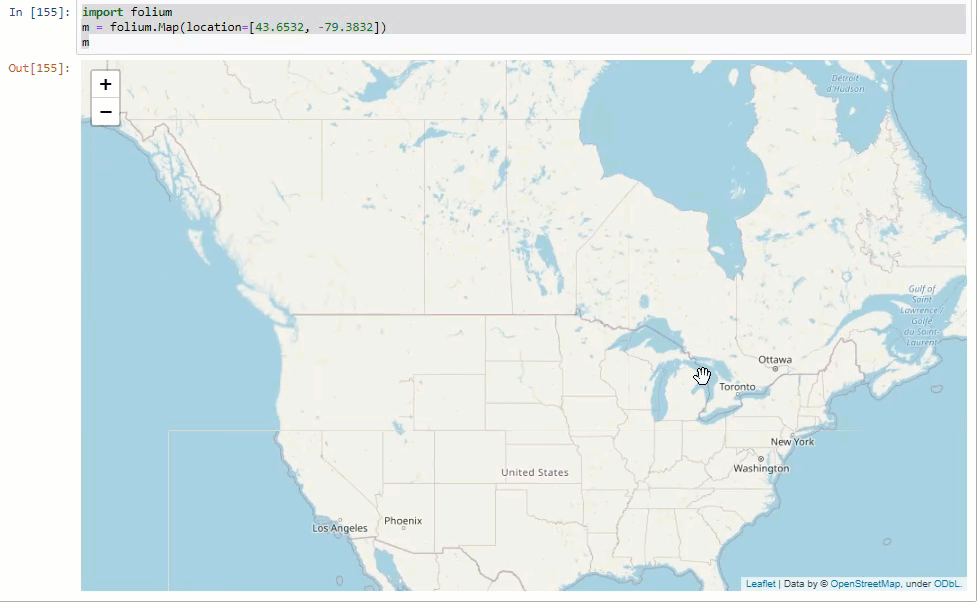OSM Map
`folium.Map(location=[43.6532, -79.3832], zoom_start=12, tiles=’https://services.arcgisonline.com/arcgis/rest/services/World_Topo_Map/MapServer/WMTS/tile/1.0.0/World_Topo_Map/default/default028mm/{z}/{y}/{x}.png', attr=’Ablajan or anything else...’)`Esri World_Topo_Map
`#load gdf to mapgjson = corona_gpd.to_crs(epsg=’4326').to_json()#embed map def embed_map(m):   from IPython.display import IFrame   m.save(‘index.html’)   return IFrame(‘index.html’, width=’100%’, height=’750px’)map = folium.Map([43.783333, -79.866667], zoom_start=2)country = folium.features.GeoJson(gjson)map.add_child(country)embed_map(map)`
`# add iframe in notebookdef embed_map(m):    from IPython.display import IFrame    m.save('index.html')    return IFrame('index.html', width='100%', height='500px')#add basemapmap = folium.Map([0, 0], zoom_start=2, tiles='https://services.arcgisonline.com/arcgis/rest/services/World_Topo_Map/MapServer/WMTS/tile/1.0.0/World_Topo_Map/default/default028mm/{z}/{y}/{x}.png', attr='Esri ..., Ablajan or anything else...')gjson = corona_gpd.to_crs(epsg='4326').to_json()df3 = df2.set_index('CNTRY_NAME')['TotalDeaths'].dropna()colorscale = branca.colormap.linear.YlOrRd_09.scale(df2.TotalDeaths.min(), df2.TotalDeaths.max())def style_function(feature):    TotalDeaths = df3.get(int(feature['id'][-1:]), None)    return {        'fillOpacity': 1,        'weight': 1,        'fillColor': '#black' if TotalDeaths is None else colorscale(TotalCases)    }colorscale.add_to(map)colorscale.caption = 'Total Deaths by Country'country = folium.features.GeoJson(gjson,                                  tooltip=folium.features.GeoJsonTooltip(fields=['TotalDeaths']),                                  style_function=style_function)map.add_child(country)folium.LayerControl().add_to(map)# save map as htmlresults =”C:\\Users\\Desktop\\map\\”map.save(os.path.join(results, ‘Total_Deaths_by_Country.html’))embed_map(map)`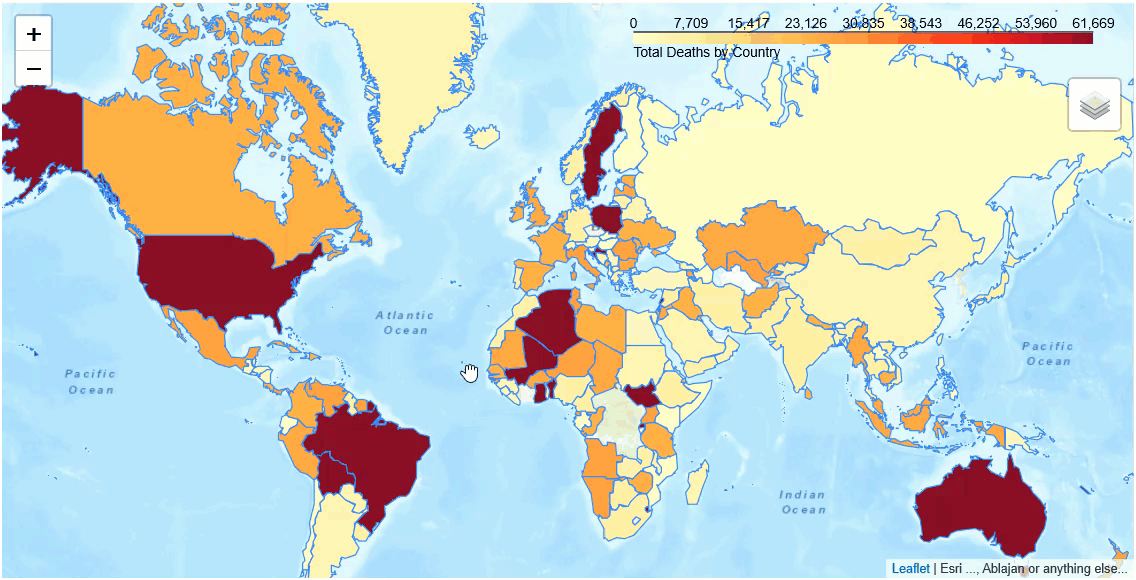COVID-19 Total Deaths by Country
`covid= pd.read_csv(‘https://covid.ourworldindata.org/data/owid-covid-data.csv') # filter certain countrycovid_ca =covid[covid.location =='Canada'].sort_values(['total_cases'],ascending=False) covid_ca.head(10)`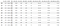COVID-19 in Canada
`# keep wanted columns onlycovid_gdp=covid_gdp[[‘COUNTRY’, ‘TotalCases’, ‘TotalDeaths’,’NewDeaths’, ‘TotalRecovered’, ‘ActiveCases’,’Serious_Critical’, ‘geometry’ ]]import keplerglcorona = keplergl.KeplerGl(height=500)corona.add_data(data=covid_gdp, name='Covid_19')corona.save_to_html(file_name='.\covid19_map.html')corona`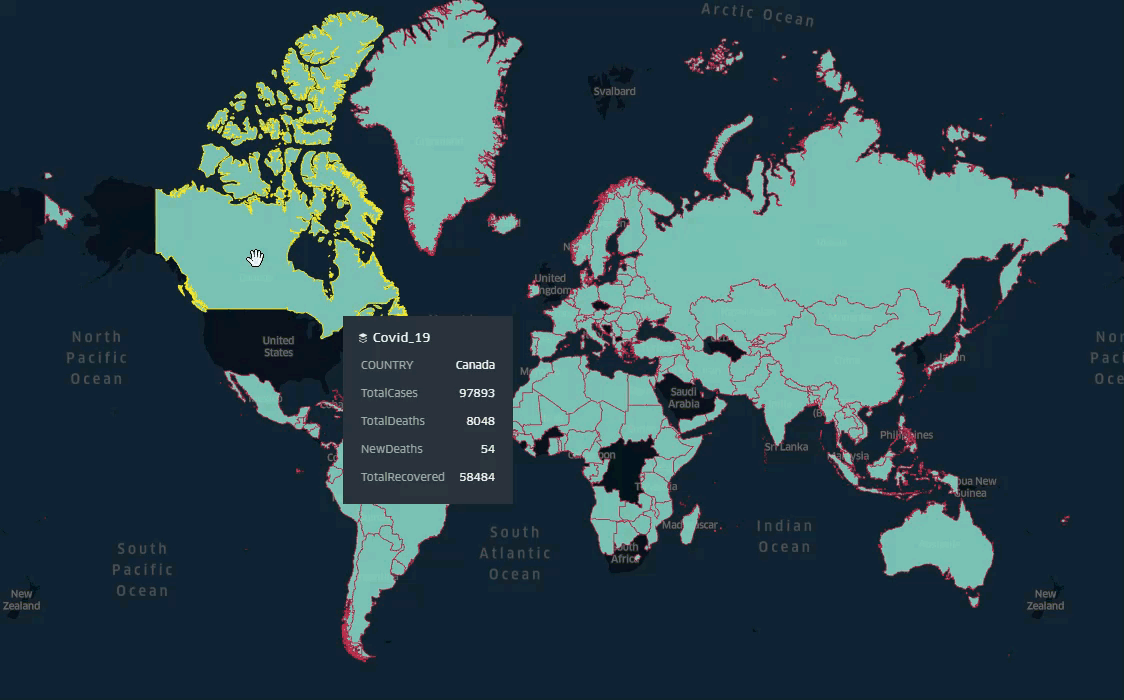kepler.gl Interactive Map

Senior Geospatial Specialist in Toronto

## More from Ablajan Sulaiman

Senior Geospatial Specialist in Toronto